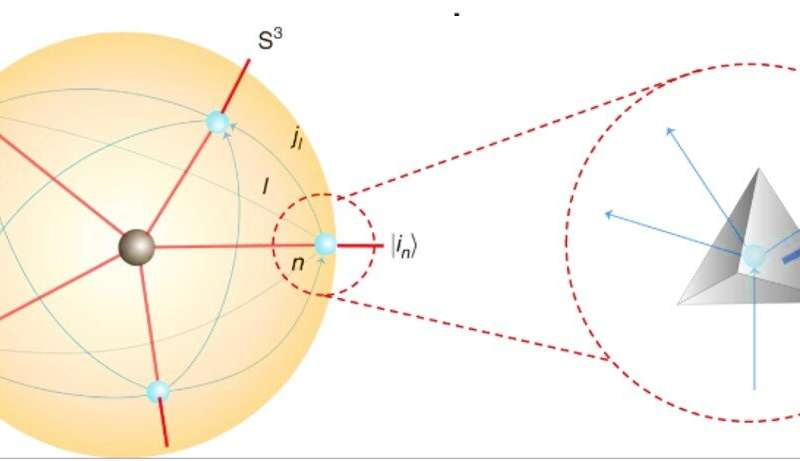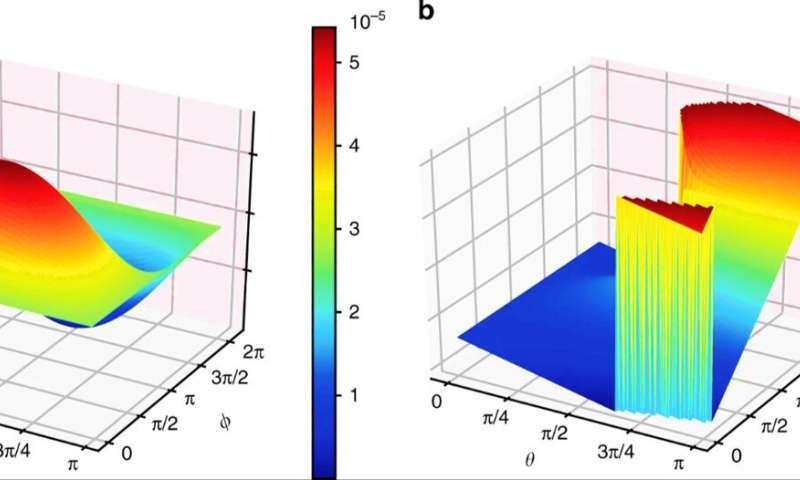OCTOBER 18, 2019 by Thamarasee Jeewandara , Phys.org

Quantum spacetime and tetrahedra. (a) A static 4d quantum spacetime from evolving the spin network. (b) A dynamical quantum spacetime with a number of five valent vertices (in black) by intersecting world sheets, one of which is denoted by S3. (c) The local structure of a vertex from b by considering a 3-sphere S3 enclosing the vertex. Intersections between the world sheets and S3 give a spin network (in blue). Each spin network represents a state |in⟩ and each link l is oriented, which carries a half-integer jl. (d) Quantum geometrical tetrahedra. Each node of the spin network represents a quantum tetrahedron. Connecting 2 nodes by a link in the spin network corresponds to gluing 2 tetrahedra through the face dual to the link. Oriented areas are denoted E(k=1,⋯,4)=(E(k)x,E(k)y,E(k)z). Credit: Communication Physics, doi: 10.1038/s42005-019-0218-5

Quantum simulation plays an irreplaceable role in diverse fields, beyond the scope of classical computers. In a recent study, Keren Li and an interdisciplinary research team at the Center for Quantum Computing, Quantum Science and Engineering and the Department of Physics and Astronomy in China, U.S. Germany and Canada. Experimentally simulated spin-network states by simulating quantum spacetime tetrahedra on a four-qubit nuclear magnetic resonance (NMR) quantum simulator. The experimental fidelity was above 95 percent. The research team used the quantum tetrahedra prepared by nuclear magnetic resonance to simulate a two-dimensional (2-D) spinfoam vertex (model) amplitude, and display local dynamics of quantum spacetime. Li et al. measured the geometric properties of the corresponding quantum tetrahedra to simulate their interactions. The experimental work is an initial attempt and a basic module to represent the Feynman diagram vertex in the spinfoam formulation, to study loop quantum gravity (LQG) using quantum information processing. The results are now available on Communication Physics.

Classical computers cannot study large quantum systems despite successful simulations of a variety of physical systems. The systematic constraints of classical computers occurred when the linear growth of quantum system sizes corresponded to the exponential growth of the Hilbert Space, a mathematical foundation of quantum mechanics. Quantum physicists aim to overcome the issue using quantum computers that process information intrinsically or quantum-mechanically to outperform their classical counterparts exponentially. In 1982, Physicist Richard Feynman defined quantum computers as quantum systems that can be controlled to mimic or simulate the behaviour or properties of relatively less accessible quantum systems.

In the present work, Li et al. used nuclear magnetic resonance (NMR) with a high controllable performance on the quantum system to develop simulation methods. The strategy facilitated the presentation of quantum geometries of space and spacetime based on the analogies between nuclear spin states in NMR samples and spin-network states in quantum gravity. Quantum gravity aims to unite the Einstein gravity with quantum mechanics to expand our understanding of gravity to the Planck scale (1.22 x 1019 GeV). At the Planck scale (magnitudes of space, time and energy) Einstein gravity and the continuum of spacetime breakdown can be replaced via quantum spacetime. Research approaches toward understanding quantum spacetimes are presently rooted in spin networks (a graph of lines and nodes to represent the quantum state of space at a certain point in time), which are an important, non-perturbative framework of quantum gravity.Quantum spacetime and tetrahedra within a spin network. Credit: Communication Physics, doi: 10.1038/s42005-019-0218-5

In 1971, physicist Roger Penrose proposed spin networks motivated by the twistor theory with subsequent applications to loop quantum gravity (LQG). The spin networks were quantum states representing fundamentally discrete quantum geometries of space at the Planck scale. In the present study, the research team represented the spin network using a graph with links and nodes colored by spin halves. For example, any node with edges corresponded to a geometry and therefore a graph containing four-valent nodes corresponded to quantum tetrahedron geometry.

The research team developed a “network” containing a number of three-dimensional (3-D) world sheets (2-D surfaces) and their intersections. They showed that each vertex where the surfaces met, led to a quantum transition that changed the spin network to represent local dynamics of quantum geometry. Much like Feynman diagrams (schematic representations of mathematical expressions describing the behavior of subatomic particles), quantum spacetimes encoded the transition amplitudes and spinfoam amplitudes between the initial and final spin networks. The quantum spacetimes and spinfoam amplitudes developed in the study provided a consistent and promising approach to quantum gravity. Li et al. featured the NMR simulation by the capability to control individual qubits with high precision. The quantum tetrahedra and vertex amplitudes served as building blocks of LQG (loop quantum gravity) to open a new window to include LQG in quantum experiments.

The scientists first derived equations to describe a quantum tetrahedron within a spin network. In a schematic 3+1-dimensional dynamic quantum spacetime model, they demonstrated an atom as a 3-sphere enclosing a portion of the quantum spacetime surrounding a vertex. The team modeled the boundary of the enclosed quantum spacetime precisely as a spin network and showed the possibility of simulating large quantum spacetimes with many vertices by quantum gluing the atoms. The resulting structure resembled vertex amplitude of quantum spacetime similar to previously developed Ooguri’s topological lattice models in four dimensions. The researchers showed LQG to identify quantum tetrahedron geometries with the quantum angular momenta. The identification allowed them to simulate quantum geometries with quantum registers (quantum mechanical analogue of a classical processor register). In general, a quantum register can be mathematically achieved using tensor products.TOP: Experimentally prepared states on the Bloch sphere and their corresponding classical tetrahedra. The states take the form cosθ2|0⟩L+eiϕsinθ2|1⟩L and are labelled by Ai, Bi, Ci, Di, Ei (i=0,1), among which, C0 and C1 are regular tetrahedrons. |0L⟩ and |1L⟩ are the basis states in a subspace of a four-qubit system, representing a single logical qubit. BOTTOM: Cosine values of angles between face normals in the quantum tetrahedron (cosines of dihedral angles differ by a minus sign). The results in experiments (theory) are represented by the colored (transparent) columns. Error bars came from the uncertainty when fitting nuclear magnetic resonance (NMR) spectra. Credit: Communication Physics, doi: 10.1038/s42005-019-0218-5

During the experiments, Li et al. simulated 10 quantum tetrahedra by preparing the corresponding invariant-tensor states. They labeled these states using 10 colored points on the Bloch sphere (geometrical representation) and conducted the experiments on a 700-MHz DRX Bruker spectrometer at room temperature. For all experiments, the research team used the crotonic acid molecule with four 13C nuclei suited for the four-qubit system. The scientists developed the experimental system to prepare quantum tetrahedra and simulate its local dynamics in three parts.

1. For state preparation, first they initialized the entire system to a pseudo-pure state. They obtained a fidelity above 99 percent using the spatial average method. Then they drove the system into 10 invariant-tensor states or transformations, which they implemented using 10 shaped pulses of 20 ms.
2. Next, for geometry measurements, the team presented the measured geometry properties using a 3-D histogram. The experimental uncertainty at this point resulted from the NMR spectrum-fitting process. The coincidence between experimental and theoretical simulations implied that the invariant tensor states prepared in the experiments matched the building blocks—quantum tetrahedra.
3. During amplitude simulation, the spin-network states served as the boundary data of 3+1-dimensional quantum spacetime. The vertex amplitude defined in the study determined the spinfoam amplitude and described the local dynamics of quantum gravity in 4-D quantum spacetime, to display the properties of these boundary data.LEFT: Structure of Crotonic Acid molecule; The four 13C nuclei are denoted as the four qubits and the table on the left presents the parameters constructing the internal Hamiltonian. Chemical shifts (Hz), J-coupling strengths (Hz) and the relaxation times( T1 and T2) are listed in the diagonal part, off-diagonal elements and the bottom, respectively. All parameters were measured on a Bruker DRX 700 MHz spectrometer at room temperature. RIGHT: Pulse sequences for creating the pseudo-pure state. Based on the spatial average technique, the circuits includes local operations, five J-coupling evolutions, and four z-gradient pulses to destroy the unwanted coherent terms. the duration of 1/2J free evolution depends on the strength of the J-coupling between relevant spins. Credit: Communication Physics, doi: 10.1038/s42005-019-0218-5

In order to obtain the vertex amplitudes, the researchers calculated the inner products between five different quantum tetrahedron states. Ideally, the researchers could have used a 20-qubit quantum computer, establishing two-qubit maximally entangled states between two arbitrary tetrahedra. However, since a quantum computer of such dimensions is presently beyond commercialized cutting-edge technology, the researchers alternately conducted full tomography of the state preparation to obtain information of quantum tetrahedron states. When the scientists calculated the fidelities between the experimental quantum tetrahedron states and theory, the results were well above 95 percent. Using the quantum tetrahedra, the research team simulated the vertex amplitude. They compared the results between the experiment and the numeric simulation among all five tetrahedra. Accordingly, saddle points of the amplitude in the experiments occurred where the five interacting tetrahedra demonstrated a simple geometric meaning as they glued to form a geometric four-simplex.Results of simulated vertex amplitudes a are the amplitude of Eq. (3) and b describe the information of its phase. θ and ϕ are the parameters of the four-qubit invariant-tensor state corresponding to the spherical coordinates on the Bloch sphere. Credit: Communication Physics, doi: 10.1038/s42005-019-0218-5

In this way, Keren Li and co-workers used a quantum register in the NMR system to create 10 invariant-tensor states to represent 10 quantum tetrahedra. They achieved a fidelity above 95 percent and subsequently measured the dihedral angles (two plane faces) of the model. They considered the spectrum-fitting errors and geometrical identification to understand the success in simulating quantum tetrahedra in the study. The new research work presented a first-step to explore spin-network states and spinfoam amplitudes using a quantum simulator. The accompanying work also demonstrated valid experiments to study LGQ.

More information: Keren Li et al. Quantum spacetime on a quantum simulator, Communications Physics (2019). DOI: 10.1038/s42005-019-0218-5

Richard P. Feynman. Simulating physics with computers, International Journal of Theoretical Physics (2007). DOI: 10.1007/BF02650179

S. Lloyd. Universal Quantum Simulators, Science (2006).
DOI: 10.1126/science.273.5278.1073
Journal information:Communications Physics Science

`Collected at:  https://phys.org/news/2019-10-quantum-spacetime-simulator.html?utm_source=nwletter&utm_medium=email&utm_campaign=weekly-nwletter `

## 2 thoughts on “Quantum spacetime on a quantum simulator”

1.ปั้มไลค์ says:

Like!! I blog frequently and I really thank you for your content. The article has truly peaked my interest.

2.plenty of fish dating site says:

Hello very cool blog!! Guy .. Excellent .. Amazing .. I’ll bookmark your website and
take the feeds also? I am happy to search out a lot of helpful
info right here in the post, we’d like develop extra techniques on this regard,
thanks for sharing. . . . . .